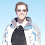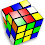## Friday, March 21, 2008

### Billiard Balls Puzzle

In the American game of "eight-ball" there are 15 numbered balls (1 through 15). At the beginning of the game, these balls are racked into a triangular pattern as shown.

The challenge this week is to place the numbers 1 through 15 into an upside-down triangle pattern such that each number is the result of *subtracting* the two numbers above it. To eliminate mirrored answers, provide a solution where the numbers at the three points of the triangle are in ascending order going clockwise.

P.S. When taking the difference, always use the absolute value. Feel free to add a comment with your answer, along with how you solved it.

1.I haven't come up with a solution yet.
It shouldn't be too hard to brute-force it with a computer program... since the 15 must go on the top line, there's only 24,000 permutations of the top line to test.

But someone's got to have a better idea!

2.6 14 15 3 13
8 1 12 10
7 11 2
4 9
5

3.I'm not sure how ericmargel got 24,000 permutations to test for the top line.

My reasoning is that there are 60,060 configurations of the top line to test. Here's the idea, there are 15 balls, the 15 must go on the top row leaving 4 choices from 14 to be made (1,001 ways). Once those have been made there are 5!=120 ways to arrange the balls along the top row. But since we're not interested in reflections, we divide by 2 since each configuration of the top row is equivalent to one other. So there are

1001 * 120 / 2 = 60,060 ways to configure the top row.

4.I'm assuming he took the 1001 ways and assumed the 15 ball was fixed, leaving 4! ways to arrange the other balls. As you pointed out, this should be 1001 times 5! since we don't know where the 15 ball should go. (Then after that you can divide by 2 for the reflection).

So I'm curious. Eric, did you use the "brute-force" method or another?

For NPR puzzle posts, don't post the answer or any hints that could lead to the answer before the deadline (usually Thursday at 3pm ET). If you know the answer, submit it to NPR, but don't give it away here.

You may provide indirect hints to the answer to show you know it, but make sure they don't assist with solving. You can openly discuss your hints and the answer after the deadline. Thank you.